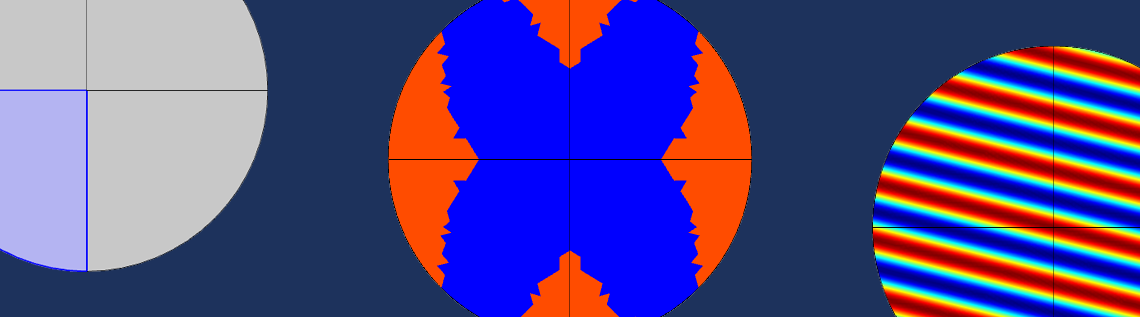# Using the Expression Operator in COMSOL Multiphysics®

November 17, 2022

The Expression Operator node is used for evaluating arbitrary parameterized expressions. You can add multiple instances of this node to one model, with each node defining separate expressions to be used on or in different geometric entities such as domains or boundaries. In this blog post, we will look at how the Expression Operator works and how you can use it in your modeling projects when working with the COMSOL Multiphysics® software.

### The Expression Operator, Explained

The Expression Operator node, introduced with version 5.5 of COMSOL Multiphysics, is available from the Variable Utilities submenu (which you activate by selecting the Variable Utilities check box under General in the Show More Options dialog box) when you right-click either the Global Definitions or Definitions node in the Model Builder. (When added under Global Definitions, the Expression Operator is valid in the entire model.) The settings for the Expression Operator node include a Definition section, in which you can define the operator. To do so, follow the instructions below.

Step 1: Type the Default Expression

In the Expression field, type the default expression that defines the expression operator on its selection (or globally when added under Global Definitions). The expression can contain any constants, variables, functions and operators that are valid in the context where the expression operator is going to be evaluated. In addition, it should make use of at least one input argument. Note that the default expression can be overridden by Operator Contribution nodes on a subselection.

Step 2: Define the Input Argument

In the table below the Expression field, define the input arguments. Type the name of the argument in the Argument column and a unit to define its dimension in the Dimensions column; in the Argument type column, choose the Expression option (the default setting) or the Value option from the drop-down list. Passing an input argument by value means that it is evaluated in the context where the expression operator is called, before being inserted as a number into the operator expression. Arguments passes by expression are substituted directly into the operator expression. This can make a difference if the argument is passed on as an argument to a coupling operator that evaluates its arguments in a different context. For example, care must be taken when passing a spatial coordinate variable in an argument that is forwarded to an Integration coupling that is part of the expression operator’s definition.

Step 3: Enter a Name

In the Name field, enter a suitable name for the expression operator. It will be the name that you will use when referring to the expression operator in the COMSOL model.

### Benefits of the Expression Operator

The Expression Operator can benefit a variety of modeling projects and scenarios. Learn how in the list below:

• Once you define the expression operator and decide on a descriptive name for it, you can use it in your COMSOL model to hide complex mathematical expressions. This means that you can instead evaluate expressions written in shorthand.
• An Expression Operator node can have different definitions in different parts of the model geometry using the Operator Contribution subnodes. You can add the Operator Contribution subnodes to the Expression Operator nodes defined under a Component node. For each such subnode, you can define the geometric entity level and the selection of geometric entities where it will be used. You can then type the new operator expression in the Expression field of the Operator Contribution subnode. This capability makes the Expression Operator node a good tool for handling difficulties in postprocessing for multilayered shell interfaces, for example. For such postprocessing it’s beneficial to be able to use a single operator that can expand to different operators on different selections. It then works as a wrapper, merging different operators and expressions on different domains or other geometric entities.
• The Expression Operator can also be used to create hybrid functions that mix arguments and variables that are evaluated locally.

### Expression Operators vs. Analytic Functions

You can think of an expression operator as a parameterized variable rather than an analytic function. Contrary to an analytic function, an expression operator can be defined to be active only in some domains. An expression operator can, in its definition, use any expression that can be evaluated where the expression operator is evaluated; an analytic function, in contrast, should be a pure function of its arguments. An analytic function can, thanks to its global nature, be used in the definition of model parameters; an expression operator cannot.

### Example of the Expression Operator in Action

This example shows how an Expression Operator node can be used for the following two purposes:

1. To substitute an expression as an argument into different operators depending on where the expression operator is evaluated. In this example, this is used to plot a double mirror symmetry.
2. To mix arguments and variables via hybrid functions, such as the operator phase(kx,ky) in this example.

In the example, a Solid Mechanics interface is only defined in the first (upper-right) quadrant of the model geometry, which is a unit circle. Two General Extrusion operators, yAxisMirror and xAxisMirror, provide mirror operations from a source to the destination for the x– and y-coordinates as –x and y and x and –y, respectively. An Expression Operator called doubleMirror is then defined using three Operator Contribution subnodes, each providing a double-mirror transformation in the second, third, and fourth circle quadrants as yAxisMirror(expr), xAxisMirror(yAxisMirror(expr)), and  xAxisMirror(expr), respectively. The following image shows the settings for the Operator Contribution subnode for the third quadrant: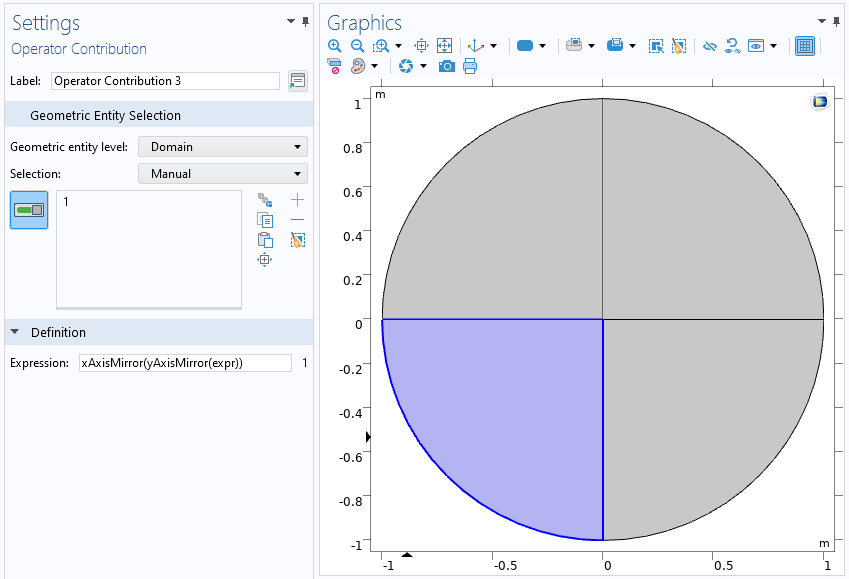The definition of the Expression Operator in the third quadrant.

The following plot shows areas where the effective von Mises stress exceeds some limit: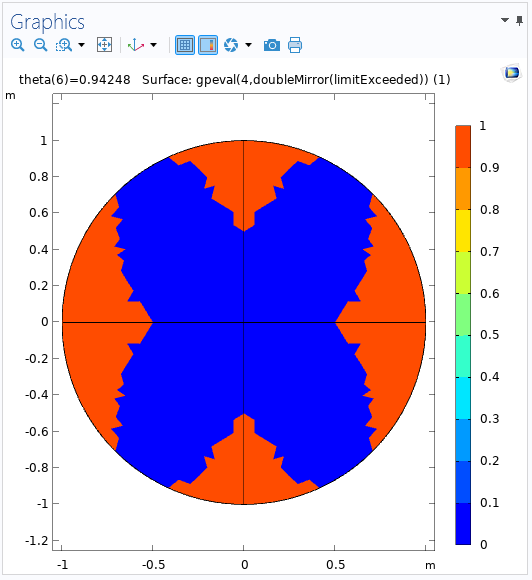Plot of areas where a stress limit has been exceeded. The double mirror provided by an Expression Operator includes a solution in the entire circle.

Thanks to the Expression Operator, the solution computed for the first quadrant can be visualized as a double-mirror plot in the entire circle with just a compact expression.

For the second type of use of the Expression Operator, an Expression Operator called phase is defined in the entire geometry and mixes arguments and variables that are evaluated locally. The Expression Operator is defined as exp(-i(kx*x+ky*y)), where kx and  ky are two input arguments, with the former representing the x-components of the wave vector and the latter representing the y-components. They are also defined as expressions, as seen in the following image of the Settings window: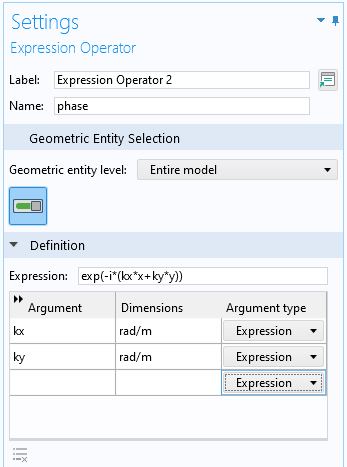The Settings window for the phase Expression Operator with the two expression arguments.

The expression for the phase is the equivalent of the mathematical expression e^{-i(k_x \cdot x+k_y \cdot y) using COMSOL Multiphysics syntax.

You can use the phase Expression Operator in a surface plot to show the phase with different values of the arguments. The following plot shows the phase with kx set to 5 and  ky set to 20: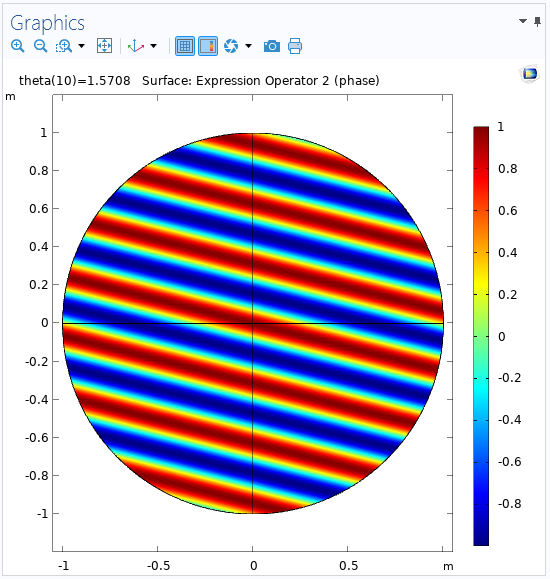A surface plot showing the phase using an Expression Operator.

### Next Steps

This blog post has explained what the Expression Operator node is and how you can use it to your advantage when modeling in COMSOL Multiphysics. We encourage you to practice using this feature to simplify and improve your COMSOL models. If you have any questions related to this topic, contact COMSOL via the button below.

Want to learn more about the Expression Operator node? Check out our blog post “Getting the Stats: Computing Standard Deviations and Other Statistical Quantities”, where the Expression Operator is used to simplify an expression for the standard deviation that is used in several places.

#### Categories##### Ruoyu Dai
December 5, 2022

Can we get the sample mph file for this tutorial?##### Magnus Ringh
December 5, 2022 COMSOL Employee

Hi Ruoyu, and thanks for your suggestion. We will make the example MPH-file available through a link from this blog post soon.

EXPLORE COMSOL BLOG